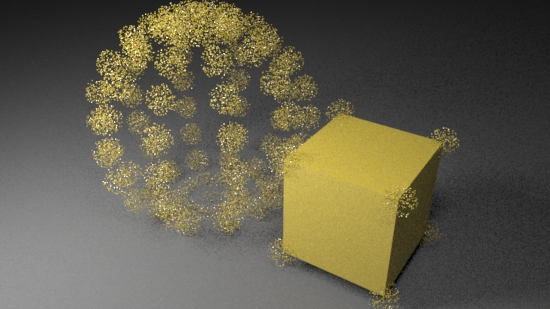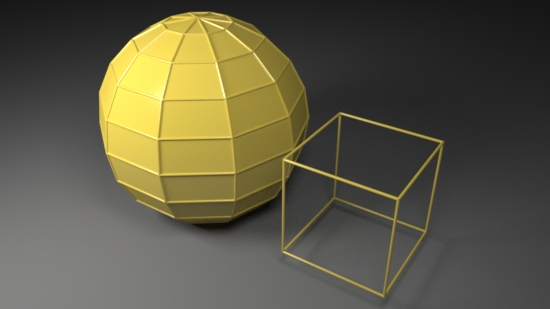### RfMPython Rifs Getting Started 2

#### Introduction

This tutorial is an extension of the tutorial "Python Rifs Getting Started 1". The reader should carefully follow that tutorial before following the instructions shown on this page.

#### Using the Vertex Data to Place a "Cluster" of Points

Select both polymesh surfaces in Maya and execute the following MEL procedure in the Add_Primvar tab of the script editor.

`addFloatPrimVar("render_mode", 1);`

Save the following script shown in listing 5 as rif_mesh_geo5.py and edit the Rif_Py tab - as shown below. Note the use of the `rif_mesh_geo5.Rif(200, 0.3, 0.02)`.

```import batch
import rif_mesh_geo5
rifs = ['rif_it','rif_mesh_geo5.Rif(250, 0.3, 0.02)' ]
batch.render(1,1, rifs)```

Listing 5 - rif_mesh_geo5.py

 ```import prman from rif_utilities import MeshDB from rif_utilities import getFloatPrimvar from rif_utilities import genPointsAroundVertex class Rif(prman.Rif): MESH = 0 POINTS = 1 POINTS_AND_MESH = 2 DEFAULT_MODE = MESH def __init__(self, ri, num, rad, width): prman.Rif.__init__(self, ri) self.num = num # number of points clustered per vertex self.rad = rad # radius of each cluster self.width = width # the size of the points in each cluster def PointsGeneralPolygons(self, nloops, nverts, verts, params): ##___________________________________ render_mode = int(getFloatPrimvar('render_mode', params, Rif.DEFAULT_MODE)) if render_mode == Rif.MESH: self.m_ri.PointsGeneralPolygons(nloops, nverts, verts, params) return mesh = MeshDB(nloops, nverts, verts, params) vertices = mesh.getVertices() vert_counter = 0 for vert in vertices: extra_points = genPointsAroundVertex(self.num, vert, self.rad, vert_counter) points_params = { prman.Ri.P : extra_points, prman.Ri.CONSTANTWIDTH : self.width } self.m_ri.Points(points_params) vert_counter += 1 if render_mode == Rif.POINTS_AND_MESH: self.m_ri.PointsGeneralPolygons(nloops, nverts, verts, params) ##___________________________________ ```

Using the new rif should produce an image similar to figure 5.Figure 5

#### Using the Vertex Data to Place a Curve around each Face

Save the following script shown in listing 6 as rif_mesh_geo6.py and edit the Rif_Py tab - as shown below. Note the use of the `rif_mesh_geo6.Rif(0.05)`.

```import batch
import rif_mesh_geo6
rifs = ['rif_it','rif_mesh_geo6.Rif(0.05)' ]
batch.render(1,1, rifs)```

Listing 6 - rif_mesh_geo6.py

 ```import prman from rif_utilities import MeshDB from rif_utilities import getFloatPrimvar class Rif(prman.Rif): MESH = 0 CURVES = 1 CURVES_AND_MESH = 2 DEFAULT_MODE = MESH def __init__(self, ri, width): prman.Rif.__init__(self, ri) self.width = width def PointsGeneralPolygons(self, nloops, nverts, verts, params): ##___________________________________ render_mode = int(getFloatPrimvar('render_mode', params, Rif.DEFAULT_MODE)) if render_mode == Rif.MESH: self.m_ri.PointsGeneralPolygons(nloops, nverts, verts, params) return mesh = MeshDB(nloops, nverts, verts, params) curves_verts = mesh.getAllLoops() curves_params = {prman.Ri.P : curves_verts, prman.Ri.CONSTANTWIDTH : self.width } self.m_ri.Curves(prman.Ri.LINEAR, nverts, prman.Ri.PERIODIC, curves_params) if render_mode == Rif.CURVES_AND_MESH: self.m_ri.PointsGeneralPolygons(nloops, nverts, verts, params) ##___________________________________ ```

Using the new rif should produce an image similar to figure 6.Figure 6

#### Using the Vertex Data to Place a Curve at each Vertex Normal

Save the following script shown in listing 7 as rif_mesh_geo7.py and edit the Rif_Py tab - as shown below. Note the use of the `rif_mesh_geo6.Rif(0.05)`.

```import batch
import rif_mesh_geo7
rifs = ['rif_it','rif_mesh_geo7.Rif(1, 0.05)' ]
batch.render(1,1, rifs)```

Listing 7 - rif_mesh_geo7.py

 ```import prman from rif_utilities import MeshDB from rif_utilities import getFloatPrimvar class Rif(prman.Rif): MESH = 0 NORMALS = 1 NORMALS_AND_MESH = 2 DEFAULT_MODE = MESH def __init__(self, ri, length, width): prman.Rif.__init__(self, ri) self.length = float(length) # length of each line representing a normal self.width = width # diameter of each line def PointsGeneralPolygons(self, nloops, nverts, verts, params): ##___________________________________ render_mode = int(getFloatPrimvar('render_mode', params, Rif.DEFAULT_MODE)) if render_mode == Rif.MESH: self.m_ri.PointsGeneralPolygons(nloops, nverts, verts, params) return verts_per_curve = [] # 2 verts per normal - base and tip curves_verts = [] # list of base and tip vertex coordinates mesh = MeshDB(nloops, nverts, verts, params) vertices = mesh.getVertices() for vert in vertices: vx,vy,vz = vert nx,ny,nz = mesh.getAveNormal(vert) curves_verts.extend(vert) # coordinates of the base of a normal curves_verts.extend( [vx + nx * self.length, vy + ny * self.length, vz + nz * self.length] ) # ditto the tip verts_per_curve.append(2) curves_params = {prman.Ri.P : curves_verts, prman.Ri.CONSTANTWIDTH : self.width } self.m_ri.Curves(prman.Ri.LINEAR, verts_per_curve, prman.Ri.NONPERIODIC, curves_params) if render_mode == Rif.NORMALS_AND_MESH: self.m_ri.PointsGeneralPolygons(nloops, nverts, verts, params) ##___________________________________ ```

Using the new rif should produce an image similar to figure 7.Figure 7